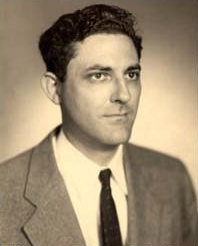# Kelly Criteria – Proof using Calculus Knowledge

## Related Post

A New Interpretation of Information Rate. Created in 1956 by John Kelly, a Bell Labs scientist.

Let us consider a communication channel which is used to transmit the results of a chance situation before those results become common knowledge, so that a gambler may still place bets at the original odds. Consider first the case of a noiseless binary channel, which might be used, for example, to transmit the results of a series of baseball games between two equally matched teams. The gambler could obtain even money bets even though he already knew the result of each game. The amount of money he could make would depend only on how much he chose to bet. How much would he bet? Probably all he had since he would win with certainty. In this case his capital would grow exponentially and after N bets he would have 2N times his original bankroll.What if there was a “w%” probability to win. How would he bet to maximize return? From a signal processing issue to risk management… It took years to come up with an answer to this question. end of 17th century both Leipniz and Newton published their books about Calculus. Newton and Leibniz  independently developed the surrounding theory of infinitesimal calculus in the late 17th century.

Original Paper : https://www.princeton.edu/~wbialek/rome/refs/kelly_56.pdf

I have created a video for the proof… It might be easier to follow… Added also the rules that might be hard to remember.

Now here is a more difficult question. What if we were winning B% of invested amount and loosing A% of invested amount. How would the Kelly Criteria look like …

Kelly % = W/A – (1 – W)/B

Simplified version Kelly % = W/A – L/B  – L:losing Percent =(1-W)

If A=B then Kelly % = W – L

VN:F [1.9.22_1171]